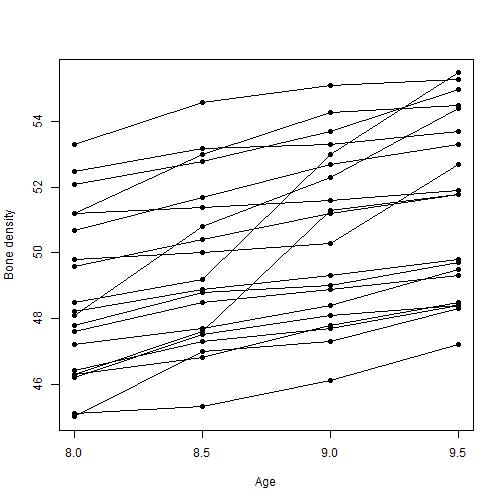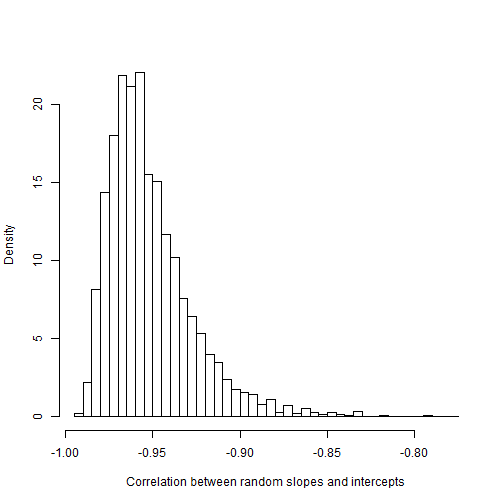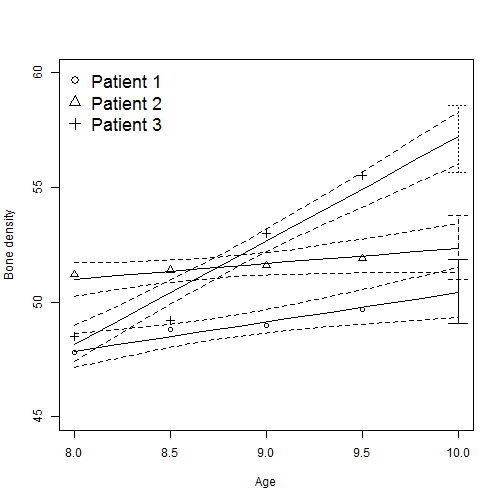# Linear mixed model for the jaw data

### Chapter 4.4: Random effects

Let $$Y_{ij}$$ be the $$j^{th}$$ measurement of jaw bone density for patient $$i$$. In this model we allow bone density to increase linearly in time and each patient has their own slope and intercept. The random slope model is $Y_{ij}|\alpha_{i1},\alpha_{i2}\sim\mbox{Normal}(\alpha_{i1}+age_j\alpha_{i2},\sigma^2)\mbox{ where }(\alpha_{1i},\alpha_{2i})^T\sim\mbox{Normal}(\mu,\Sigma).$

The random effects $$\alpha_{i1}$$ and $$\alpha_{i2}$$ are the subject-specific intercept and slope, respectively. The population of intercepts and slopes is assumed to be bivariate normal with mean vector $$\mu$$ and covariance matrix $$\Sigma$$.

The objectives are to borrow strength across patients to estimate each patient's linear trend and then predict future jaw bone density.

## Load and plot the data

 library(rjags)

m   <- 4
n   <- 20
age <- c(8.0, 8.5, 9.0, 9.5)
Y   <- c(47.8, 48.8, 49.0, 49.7,
46.4, 47.3, 47.7, 48.4,
46.3, 46.8, 47.8, 48.5,
45.1, 45.3, 46.1, 47.2,
47.6, 48.5, 48.9, 49.3,
52.5, 53.2, 53.3, 53.7,
51.2, 53.0, 54.3, 54.5,
49.8, 50.0, 50.3, 52.7,
48.1, 50.8, 52.3, 54.4,
45.0, 47.0, 47.3, 48.3,
51.2, 51.4, 51.6, 51.9,
48.5, 49.2, 53.0, 55.5,
52.1, 52.8, 53.7, 55.0,
48.2, 48.9, 49.3, 49.8,
49.6, 50.4, 51.2, 51.8,
50.7, 51.7, 52.7, 53.3,
47.2, 47.7, 48.4, 49.5,
53.3, 54.6, 55.1, 55.3,
46.2, 47.5, 48.1, 48.4,
46.3, 47.6, 51.3, 51.8)

Y <- matrix(Y,20,4,byrow=TRUE)

plot(NA,xlim=range(age),ylim=range(Y),xlab="Age",ylab="Bone density")
for(i in 1:n){
lines(age,Y[i,])
points(age,Y[i,],pch=19)
}## Put the data in JAGS format

 data     <- list(Y=Y,age=age,n=n,m=m)
burn     <- 10000
n.iter   <- 20000
thin     <- 10
n.chains <- 2


## Fit the random slopes model

 model_string <- textConnection("model{
# Likelihood
for(i in 1:n){for(j in 1:m){
Y[i,j] ~ dnorm(alpha[i,1]+alpha[i,2]*age[j],taue)
}}

# Random effects
for(i in 1:n){alpha[i,1:2] ~ dmnorm(mu[1:2],Omega[1:2,1:2])}

# Priors
for(j in 1:2){mu[j] ~ dnorm(0,0.0001)}
taue ~ dgamma(0.1,0.1)
Omega[1:2,1:2] ~ dwish(R[,],2.1)

R[1,1]<-1/2.1
R[1,2]<-0
R[2,1]<-0
R[2,2]<-1/2.1
}")

params  <- c("mu","alpha","taue","Omega")
model   <- jags.model(model_string,data = data, n.chains=n.chains,quiet=TRUE)
update(model, burn, progress.bar="none")
samples <- coda.samples(model, variable.names=params,
n.iter=n.iter, thin=thin, progress.bar="none")
samples <- rbind(samples[],samples[])
Omega   <- samples[,1:4]
a1      <- samples[,5:24]
a2      <- samples[,25:44]
mu      <- samples[,45:46]
sig     <- 1/sqrt(samples[,47])
S       <- Omega
for(i in 1:nrow(S)){
S[i,]<-as.vector(solve(matrix(Omega[i,],2,2)))
}

r <- S[,2]/sqrt(S[,1]*S[,4])
hist(r,breaks=50,prob=TRUE,main="",xlab="Correlation between random slopes and intercepts")## Make predictions

This produces the estimated (posterior median) linear trend (solid line) and 95% interval (dashed lines) for three patients, plotted against their observations (points). The vertical boxplots give the posterior predictive distribution for the measurement that will be taken at age 10. This final prediction distribution accounts for both uncertainy in the random effects $$\alpha_{ij}$$ but also measurement variance $$\sigma^2$$.

 these  <- c(1,11,12) # pick three subjects
na     <- 10
ages   <- seq(8,10,length=na) # Estimate the line for these ages

plot(NA,xlim=range(ages),ylim=c(45,60),xlab="Age",ylab="Bone density")
for(sub in 1:length(these)){

# Plot the posterior of the mean alpha1+age[j]*alpha2

i   <- these[sub]
fit <- NULL
for(j in 1:na){fit <- cbind(fit,a1[,i]+ages[j]*a2[,i])}
q  <- apply(fit,2,quantile,c(0.025,0.5,0.975))
points(age,Y[i,],pch=sub)
lines(ages,q[1,],lty=2)
lines(ages,q[2,],lty=1)
lines(ages,q[3,],lty=2)

# Plot the posterior predictive distribution at age 10

Y10 <- a1[,i]+a2[,i]*10+rnorm(length(sig),0,sig)
q   <- quantile(Y10,c(0.025,0.975))
lines(c(10,10),q,lty=sub)
lines(10+0.05*c(-1,1),rep(q,2),lty=sub)
lines(10+0.05*c(-1,1),rep(q,2),lty=sub)
}

legend("topleft",paste("Patient",1:3),pch=1:3,cex=1.5,bty="n")